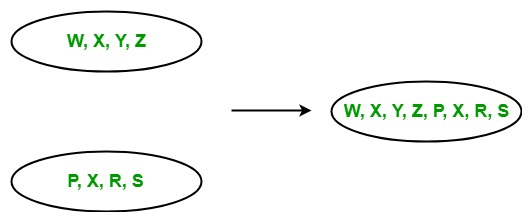Related Articles
LINQ | Concatenation Operator | Concat
• Last Updated : 22 May, 2019

The concatenation is a process in which one sequence is appended into another sequence. In LINQ, the concatenation operation contains only one operator that is known as Concat. It is used to append two same types of sequences or collections and return a new sequence or collection.

• It does not support query syntax in C# and VB.NET languages.
• It support method syntax in both C# and VB.NET languages.
• It present in both the Queryable and Enumerable class.
• It is implemented by using deferred execution.
• It allow duplicate elements.

As shown in the below image, here two sequences of the same types are concatenated into one sequence.Example 1:

 `// C# program to concatenate the``// given sequences``using` `System;``using` `System.Linq;`` ` `class` `GFG {`` ` `    ``static` `public` `void` `Main()``    ``{`` ` `        ``// Data source``        ``char``[] sequence1 = {``'p'``, ``'q'``, ``'r'``, ``'s'``, ``'y'``, ``'z'``};``        ``char``[] sequence2 = {``'p'``, ``'m'``, ``'o'``, ``'e'``, ``'c'``, ``'z'``};`` ` `        ``// Display the sequences``        ``Console.WriteLine(``"Sequence 1 is: "``);`` ` `        ``foreach``(``var` `s1 ``in` `sequence1)``        ``{``            ``Console.WriteLine(s1);``        ``}`` ` `        ``Console.WriteLine(``"Sequence 2 is: "``);`` ` `        ``foreach``(``var` `s2 ``in` `sequence2)``        ``{``            ``Console.WriteLine(s2);``        ``}`` ` `        ``// Concatenate the given array``        ``// Using Concat function``        ``var` `result = sequence1.Concat(sequence2);`` ` `        ``Console.WriteLine(``"New Sequence:"``);``        ``foreach``(``var` `val ``in` `result)``        ``{``            ``Console.WriteLine(val);``        ``}``    ``}``}`
Output:

```Sequence 1 is:
p
q
r
s
y
z
Sequence 2 is:
p
m
o
e
c
z
New Sequence:
p
q
r
s
y
z
p
m
o
e
c
z
```

Example 2:

 `// C# program to print the major ``// language of the employees``using` `System;``using` `System.Linq;``using` `System.Collections.Generic;`` ` `// Employee details``public` `class` `Employee1 {`` ` `    ``public` `int` `emp_id1``    ``{``        ``get``;``        ``set``;``    ``}`` ` `    ``public` `string` `emp_name1``    ``{``        ``get``;``        ``set``;``    ``}``    ``public` `string` `emp_lang1``    ``{``        ``get``;``        ``set``;``    ``}``}`` ` `// Employee details``public` `class` `Employee2 {`` ` `    ``public` `int` `emp_id2``    ``{``        ``get``;``        ``set``;``    ``}`` ` `    ``public` `string` `emp_name2``    ``{``        ``get``;``        ``set``;``    ``}``    ``public` `string` `emp_lang2``    ``{``        ``get``;``        ``set``;``    ``}``}`` ` `class` `GFG {`` ` `    ``// Main method``    ``static` `public` `void` `Main()``    ``{``        ``List emp1 = ``new` `List() {`` ` `            ``new` `Employee1() {emp_id1 = 209, emp_name1 = ``"Anjita"``,``                                               ``emp_lang1 = ``"C#"``},`` ` `            ``new` `Employee1() {emp_id1 = 210, emp_name1 = ``"Soniya"``,``                                                ``emp_lang1 = ``"C"``},`` ` `            ``new` `Employee1() {emp_id1 = 211, emp_name1 = ``"Rohit"``,``                                            ``emp_lang1 = ``"Java"``},`` ` `        ``};`` ` `        ``List emp2 = ``new` `List() {`` ` `            ``new` `Employee2() {emp_id2 = 219, emp_name2 = ``"Anita"``,``                                           ``emp_lang2 = ``"Scala"``},`` ` `            ``new` `Employee2() {emp_id2 = 223, emp_name2 = ``"Manya"``,``                                          ``emp_lang2 = ``"Python"``},`` ` `            ``new` `Employee2() {emp_id2 = 266, emp_name2 = ``"Rohan"``,``                                            ``emp_lang2 = ``"Ruby"``},`` ` `        ``};`` ` `        ``// Query to concatenate the major``        ``// languages of the employees of ``        ``// two different departments``        ``// Using Concat method``        ``var` `res = emp1.Select(e => e.emp_lang1).Concat(emp2.Select(e => e.emp_lang2));`` ` `        ``Console.WriteLine(``"Major Languages Used are: "``);``         ` `        ``foreach``(``var` `val ``in` `res)``        ``{``            ``Console.WriteLine(val);``        ``}``    ``}``}`
Output:
```Major Languages Used are:
C#
C
Java
Scala
Python
Ruby
```

Attention reader! Don’t stop learning now. Get hold of all the important DSA concepts with the DSA Self Paced Course at a student-friendly price and become industry ready.

My Personal Notes arrow_drop_up